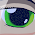## Wednesday, December 25, 2013

### Problem of the year 2014.

So it's almost 2014 (in case anyone didn't notice).

One of my favorite new year activities is the problem of the year. So as not to spoil the fun I'm going to use other years to explain

The goal is to construct the natural numbers using the digits of the year. You need to use digits in order and can use plus, minus,times,divide, sqrt, concatonation, exponentiation and factorial.

for example a list with 1997 might start like this.

1^997=1
1^9*(9-7)=2
1^9+(9-7)=3

If it was 2005 then 2+0+sqrt(0!+5!)=13 would be allowed.  In 2025 20+sqrt(25)=25 would be allowed.

You're also allowed to use cube roots if you can find a 3. e.g. (2+0!)radical(27)=3.  would be ok in 2027. because we often write cube root like a square root except with a little 3 (yes I need to figure out how to insert equations properly into this format).

Anyway let'ssee how long an unbroken list we can make for 2014 collaboratively.

1.*concatenation

1.I should probably change that.
2*0+1^4=1
2+(0*1*4)=2
2+0+1^4=3
(2*0*1)+4=4
2*0+1+4=5

2.2+0*1+4=6
2+0+1+4=7
2+0!+1+4=8
(2+0!)!-1+4=9
(2+0!)!*1+4=10
(2+0!)!+1+4=11
(2+0!)!*sqrt(1*4)=12
I can't see how to do 13 without using an initial minus

1.2+sqrt(0!+(1+4)!)=13 very proud of this one

It's fairly straightforward from 14 through 32 (unless of course I've made a mistake somewhere)
2*0+14
2-0!+14=15
2+0+14=16
2+0!+14=17
20-sqrt(1*4)=18
20-1^4=19
20*(1^4)=20
20+(1^4)=21
20+sqrt(1*4)=22
20-1+4=23
(20*1)+4=24
20+1+4=25
2+(0*1)+4!=26
2+0+1+4!=27
2+0!+1+4!=28
(2+0!)!-1+4!=29
(2+0!)!+1*(4!)=30
(2+0!)!+1+4!=31
2^(0+1+4)=32

33 has me stumped for now